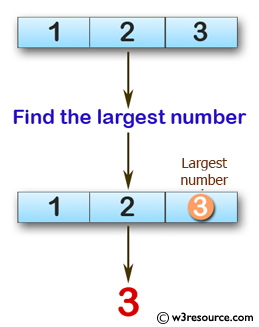﻿ Swift Basic Programming Exercise: Find the largest number among three given integers - w3resource# Swift Basic Programming Exercise: Find the largest number among three given integers

## Swift Basic Programming: Exercise-14 with Solution

Write a Swift program to find the largest number among three given integers.

Pictorial Presentation:Sample Solution:

Swift Code:

``````func max_three(_ x: Int, _ y: Int, _ z: Int) -> Int {
if x > y, x > z
{
return x
}
else if y > z, y > x
{
return y
}
else if z > y, z > x
{
return z
}
else if x == y, y > z
{
return x
}
else if y == z, z > x
{
return y
}
else
{
return x
}
}

print(max_three(1, 2, 3))
print(max_three(3, 2, 1))
print(max_three(-3, -2, 0))
```
```

Sample Output:

```3
3
0
```

Swift Programming Code Editor:

Improve this sample solution and post your code through Disqus

What is the difficulty level of this exercise?

﻿